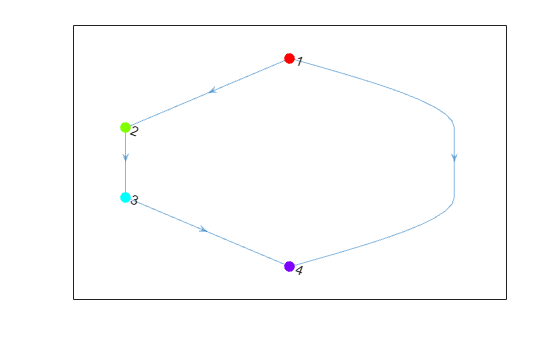# condensation

Graph condensation

## Syntax

``C = condensation(G)``

## Description

example

````C = condensation(G)` returns a directed graph `C` whose nodes represent the strongly connected components in `G`. This reduction provides a simplified view of the connectivity between components.```

## Examples

collapse all

Create and plot a graph that contains several strongly connected components. Highlight the strongly connected components.

```s = [1 1 2 3 3 4 4 4 4 5 5 6 6 6 7 8 8 9 9 10 10 10 11 11 12 13 13 14 15]; t = [1 3 1 2 5 1 2 12 13 6 8 7 8 10 10 9 10 5 11 9 11 14 12 14 13 11 15 13 14]; G = digraph(s,t); p = plot(G);``````bins = conncomp(G); p.MarkerSize = 7; p.NodeCData = bins; colormap(hsv(4))```Use `condensation` to represent each component as a single node. Color the nodes based on the components they represent.

```C = condensation(G); p2 = plot(C); p2.MarkerSize = 7; p2.NodeCData = 1:4; colormap(hsv(4))```## Input Arguments

collapse all

Input graph, specified as a `digraph` object. Use `digraph` to create a directed graph object.

Example: `G = digraph([1 2],[2 3])`

## Output Arguments

collapse all

Condensation graph, returned as a `digraph` object. `C` is a directed acyclic graph (DAG), and is topologically sorted. The node numbers in `C` correspond to the bin numbers returned by `conncomp`.

`condensation` determines the nodes and edges in `C` by the components and connectivity in `G`:

• `C` contains a node for each strongly connected component in `G`.

• `C` contains an edge between node `I` and node `J` if there is an edge from any node in component `I` to any node in component `J` of `G`.